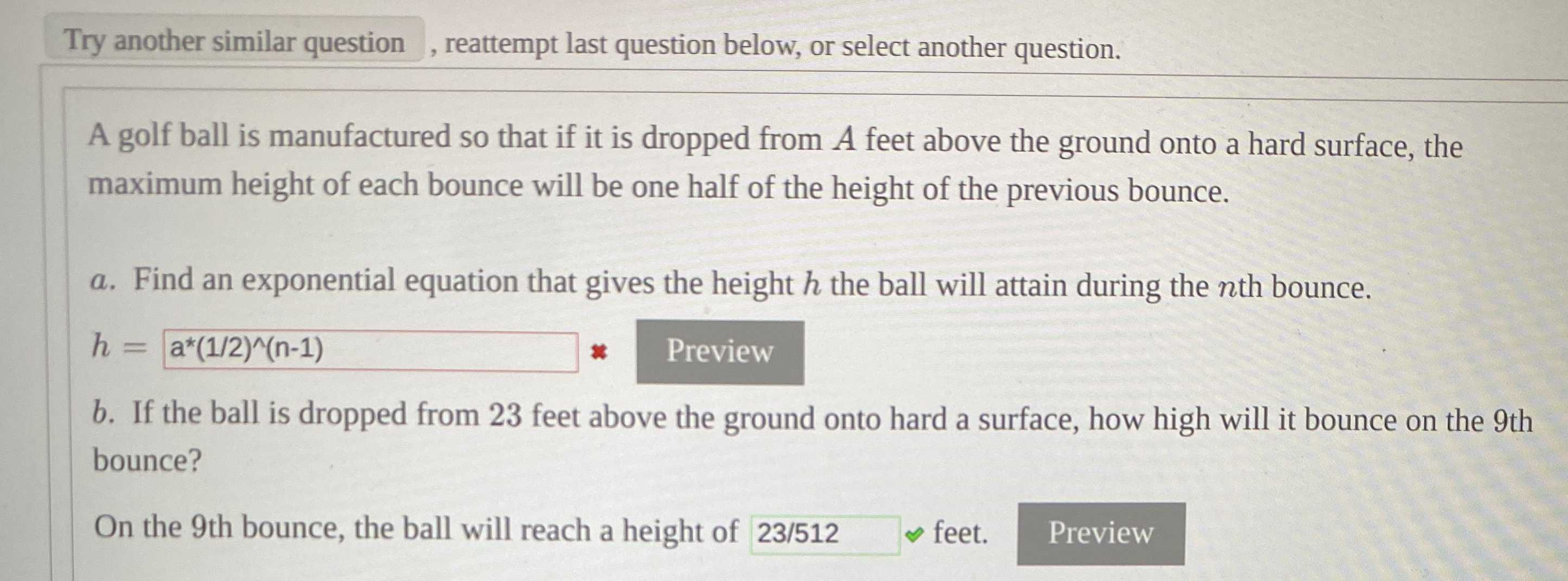### ¿Todavía tienes preguntas de matemáticas?

Pregunte a nuestros tutores expertos
Algebra
PreguntaA golf ball is manufactured so that if it is dropped from $$A$$ feet above the ground onto a hard surface, the maximum height of each bounce will be one half of the height of the previous bounce.

$$a$$ . Find an exponential equation that gives the height $$h$$ the ball will attain during the $$n$$ th bounce.

$$h = a ^ { \star } ( 1 / 2 ) ^ { \wedge } ( n - 1 )$$ $$b$$ . If the ball is dropped from $$23$$ feet above the ground onto hard a surface, how high will it bounce on the $$9$$ th bounce? On the $$9$$ th bounce, the ball will reach a height of $$23 / 512$$ feet. Preview

$$(\frac{1}{2}) ^{n}$$
$$\frac{23}{512}$$